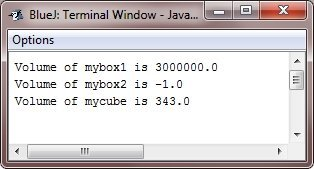In Java, you can also overload the constructor methods. In fact, for almost all real world classes that you create, overloaded constructors will be norm, not the exception. To realize why, let's return to the Box class developed in the previous chapter. Here is the latest version of the Box :

```class Box
{
double width;
double height;
double depth;

/* this is the constructor for the Box */
Box(double wid, double hei, double dep)
{
width = wid;
height = hei;
depth = dep;
}

/* compute and return the volume */
double volume()
{
return width * height * depth;
}
}```

As you can see that the Box() constructor requires the three parameters. It means that all the declarations of the Box objects must pass the three arguments to the Box() constructor. For instance, here the below statement is currently invalid :

`Box ob = new Box();`

Since Box() requires the three arguments, therefore it's an error to call without them. This raises some important questions which can be:

• What if you just wanted a box and didn't know what its initial dimensions were ?
• What if you want to be able to initialize a cube by determining only one value that would be used for all the three dimensions ?

As the Box class is currently written, these options are not available to you.

The solution to these problems is quite easy, just overload the Box constructor so that it handles the situations that described. Here is a program that contains an improved version of the Box that does just that :

```/* Java Program Example - Java Constructor Overloading
* In this program, Box defines the three constructors to
* initialize the dimensions of a box various ways.
*/

class Box
{
double width;
double height;
double depth;

/* constructor used when all the dimensions specified */
Box(double wid, double hei, double dep)
{
width = wid;
height = hei;
depth = dep;
}

/* constructor used when no dimensions specified */
Box()
{
width = -1;     // use -1 to indicate
height = -1;    // an uninitialized
depth = -1;     // box
}

/* constructor used when the cube is created */
Box(double len)
{
width = height = depth = len;
}

/* compute and return the volume */
double volume()
{
return width * height * depth;
}
}

{
public static void main(String args[])
{
/* create boxes using the various constructors */
Box mybox1 = new Box(100, 200, 150);
Box mybox2 = new Box();
Box mycube = new Box(7);

double vol;

/* get the volume of the first box */
vol = mybox1.volume();

/* print the volume of the first box */
System.out.println("Volume of mybox1 is " + vol);

/* get the volume of the second box */
vol = mybox2.volume();

/* print the volume of the second box */
System.out.println("Volume of mybox2 is " + vol);

/* get the volume of the cube */
vol = mycube.volume();

/* print the volume of the cube */
System.out.println("Volume of mycube is " + vol);
}
}```

When the above Java program is compile and executed, it will produce the following output:As you can see that the proper overloaded constructor is called based upon the parameters specified when new is executed.

Tools
Calculator